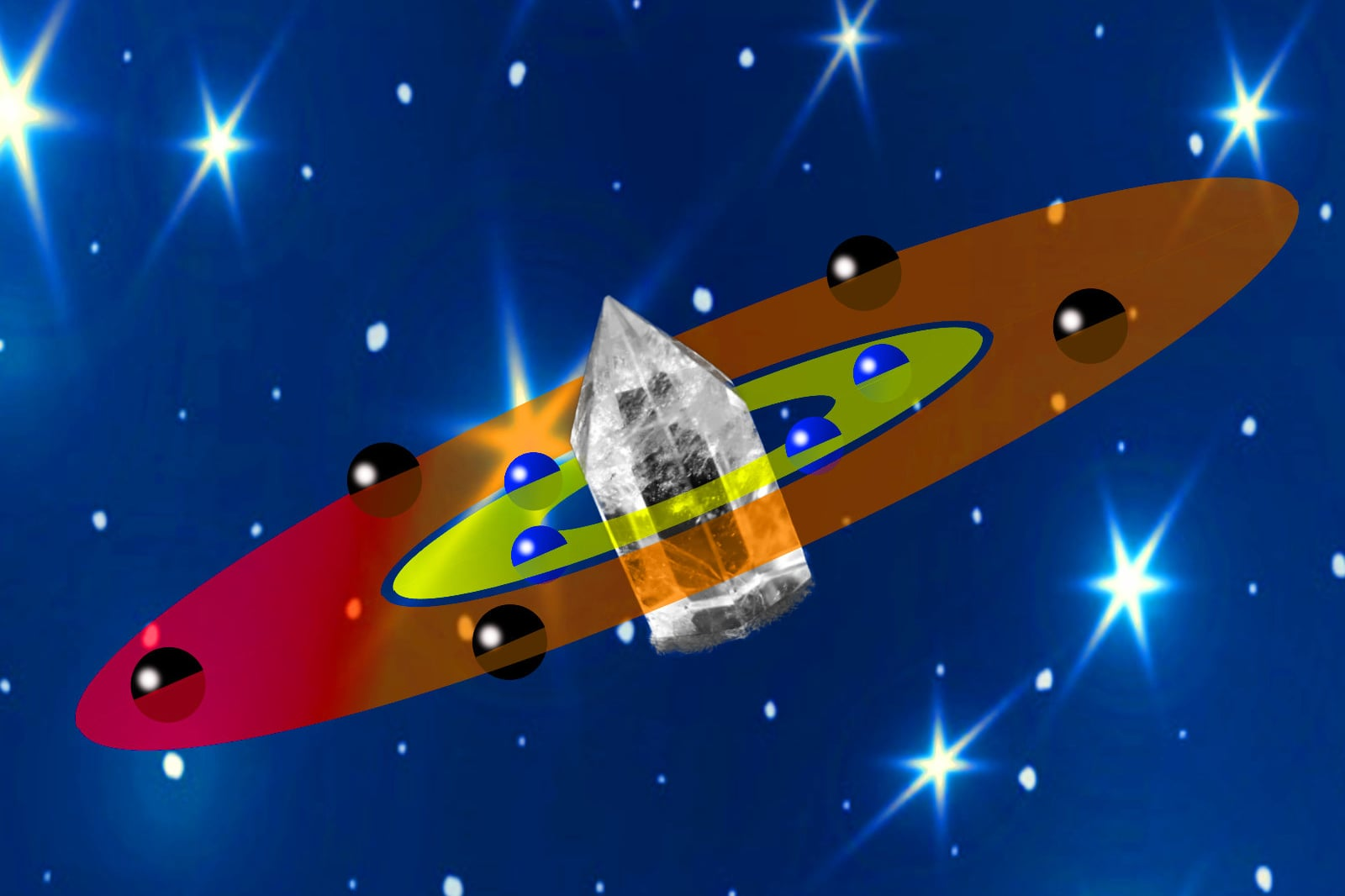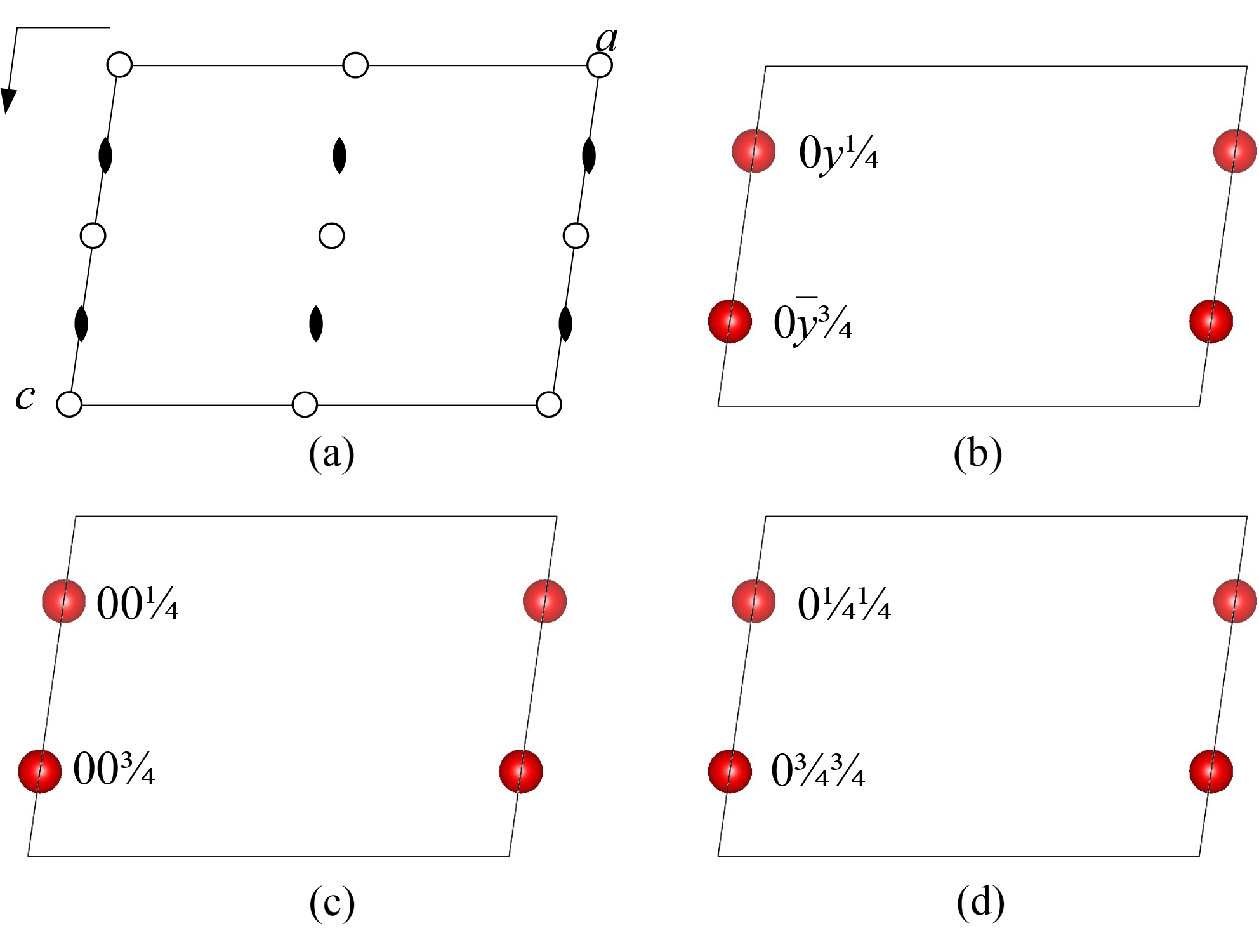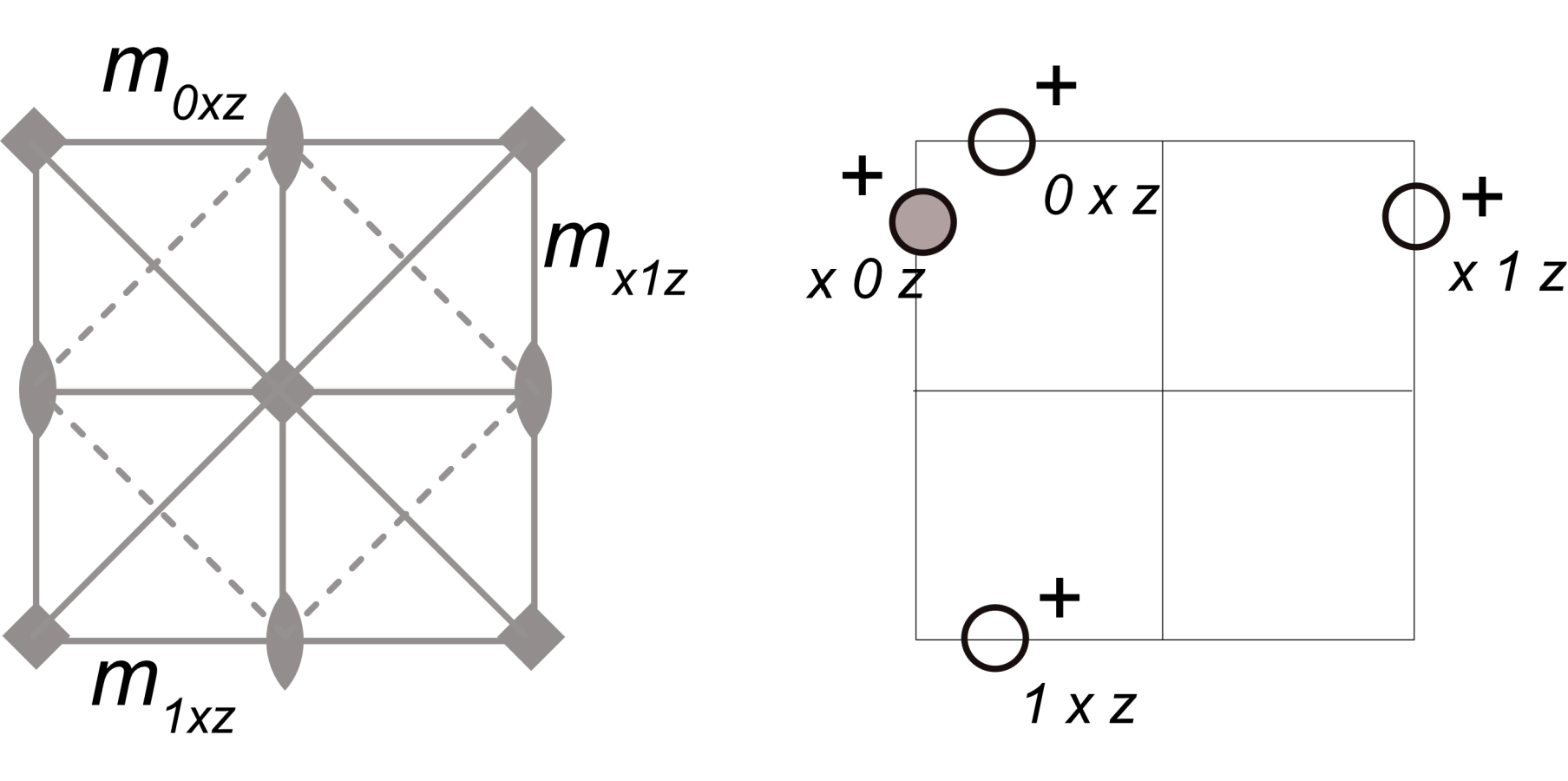•# Not so elementary, my dear Wyckoff

Massimo NespoloAmong the terms that every crystallographer uses on a daily basis, “Wyckoff position” is undoubtedly one of the most common. Anyone who has ever solved and published a crystal structure will be familiar with the “Wyckoff letter”, a handy shortcut to identify “something” in a given (type of) space group. In a world where it has become imperative to save space and time, it is indeed an obvious choice to say that a certain atom “is in position 2e of P2/c with y = 0.4125”. This is a shortcut for “an infinite set of equivalent atoms with coordinates 0,0.4125,½ and the equivalent coordinates obtained through the operations of P2/c”. But what is a Wyckoff position? Few readers may actually have ever considered such a question, and they would most likely not be able to provide a rigorous answer.

## Classifying crystals

In our daily work as crystallographers, we constantly classify crystals according to different criteria. Choosing a feature shared by different crystals allows us to put those crystals in a class. We actually use quite a number of classes, and to avoid complicated and longish titles, we label some of the classes in different ways: systems, families, etc. We say that all crystals with the same type of point group belong to the same geometric crystal class, all crystals with the same type of point group and the same type of lattice (P, I, F, C, etc.) belong to the same arithmetic crystal class, all crystals with the same morphological symmetry are in the same crystal system, all crystals with the same lattice symmetry are in the same lattice system, all crystals with the same type of conventional unit cell stay in the same crystal family, and so on.1 All these categories are indeed classes in mathematical terms, i.e. a collection of objects (concrete or abstract) that can be unambiguously defined by a property shared by all its members. Wyckoff positions are classes of crystallographic orbits. The latter term is much less known, yet it is much simpler to define and more fundamental.

## You use them but you do not name them: crystallographic orbits

Consider a crystal and forget all its defects, including the surface; in other words, describe your crystal as ideal. Next, consider an atom somewhere in the unit cell of that ideal crystal. It has an infinite number of equivalent atoms that are obtained by applying the symmetry operations of the space group (they are infinite in number: don’t forget the translations!). This infinite set of equivalent atoms is called a crystallographic orbit. It is really that simple. Then why is the name so unfamiliar? Most likely because it was introduced quite late.2 Crystallographic orbits are classified according to two criteria:

1. The eigensymmetry (inherent symmetry) of the orbit, i.e. the group of operations that leave invariant the orbit as a whole (exchanging, in general, atoms belonging to the same orbit). This eigensymmetry can never be lower than a subgroup of the space group (otherwise that orbit could not be realized in that space group), but can be higher (a supergroup). The class of crystallographic orbits whose eigensymmetry coincides with the space group leads to the name characteristic orbits. The class of crystallographic orbits whose eigensymmetry is higher than the space group is referred to as non-characteristic orbits. For instance, in our example of P2/c (Figure 1a), the crystallographic orbit generated by an atom at 0,y,¼ with y different from 0 or ¼ or ½ or multiples, coincides with the space group (Figure 1b) and is therefore a characteristic orbit. However, for y = 0 or ½ the eigensymmetry is P2/m with a half translation along c (Figure 1c), whereas for y = ¼ or ¾ it is A2/m (Figure 1d); these orbits are therefore non-characteristic orbits.Figure 1. (a) Symmetry elements in P2/c. (b) An atom placed at 0,y,¼ with y ≠ 0, ¼, ½ or multiples generates a characteristic orbit. (c) An atom placed a 0,0,¼ generates a non-characteristic orbit with eigensymmetry P2/m. (d) An atom placed at 0,¼,¼ generates a non-characteristic orbit with eigensymmetry A2/m.

2. The site-symmetry groups of the positions belonging to the crystallographic orbit. The operations of the space group that leave a position fixed in space form a group that is called the site-symmetry group of that position. Wyckoff positions are simply classes of crystallographic orbits based on site-symmetry groups.

## Site-symmetry groups and Wyckoff positions

Let us consider a position in the crystal, identified by its coordinates x1,y1,z1. Among the operations of the space group, some leave this position fixed: at least the identity operation, but often some other operations as well. If we gather all the operations that leave x1,y1,z1 fixed, we obtain a group that is called the site-symmetry group of the position x1,y1,z1. Let us call it S1; it is obviously a subgroup of the space group. If we now fix our attention on another different position with coordinates x2,y2,z2, its site-symmetry group will be indicated by S2.

The relationship between S1 and S2 provides the criterion to classify crystallographic orbits; the result is classes of crystallographic orbits known as Wyckoff positions. The relationship between S1 and S2 is the basis for the classification of crystallographic orbits:

1. S1 = S2 = 1, i.e. the site-symmetry group contains only the identity. The two positions x1,y1,z1 and x2,y2,z2, as well as all the other symmetry-related positions (xj,yj,zj), belong to a class of crystallographic orbits referred to as general because none of the operations of the space group, apart from the identity, fixes any of these positions. A different set of coordinates (x'j,y'j,z'j) defining another different and independent crystallographic orbit again has the site-symmetry group S' = 1 and so belongs to the same class. This class is known as the general Wyckoff position.

2. S1 = gS2g–1 > 1, where g is a symmetry operation of the space group G. The relationship between S1 and S2 is known as conjugation and is a similarity relation. S1 and S2 are known as conjugate subgroups of G. Conjugation can be pictorially described as “do the same somewhere else”. Two site-symmetry groups that are conjugate subgroups of the same space group contain operations of the same kind but at least some of the symmetry elements are located or oriented differently in space. Remember that a crystallographic orbit contains an infinite number of positions (xj,yj,zj) that are located differently in space. They cannot have the same site-symmetry group (with the obvious but trivial exception of the general position) but because they are symmetry-related, their site-symmetry groups are also tightly related: they are indeed conjugate. Now, all the crystallographic orbits whose site-symmetry groups are conjugate subgroups of the space group belong to the same class of crystallographic orbits, and are called special Wyckoff positions.

3. S1 and S2 are not conjugate subgroups of the space group G. The corresponding crystallographic orbits belong to different classes, i.e. to different Wyckoff positions.

Let us consider the example of space group P4mm. A position with coordinates x,0,z is left invariant by the identity operation and by a reflection through a mirror plane perpendicular to  and passing through y = 0, so that its site-symmetry group has order 2 and is denoted symbolically by .m. By applying the infinitely many symmetry operations of P4mm to x,0,z one gets infinite positions, with coordinates x,1,z; –x,0,z; –x,1,z; 0,x,z; 0,–x,z; 1,x,z; –1,–x,z and so on. Let us consider the position with coordinates 0,x,z. It is left invariant by the identity and by a reflection through a mirror plane perpendicular to  and passing through x = 0, so that its site-symmetry group has order 2 and is again symbolically indicated as .m. Now, the site-symmetry groups of the two positions are not identical: the reflection is performed through two mirror planes oriented differently. However, they are conjugate subgroups of P4mm. Indeed, by applying, for example, a fourfold rotation about  through the origin to x,0,z, one obtains 0,x,z. Formally, the site-symmetry group {1, mx0z} becomes {1, m0xz} under conjugation:

400z{1, mx0z}4–100z = {1, m0xz}.

If we choose another operation of P4mm we get a different position with a different site-symmetry group, which is again conjugate to {1, mx0z}. In the following table a few examples are explicitly shown.

 Operation Position Site-symmetry group 400z 0xz {1, m0xz} t(010) x1z {1, mx1z} 4½½z 1xz {1, m1xz}Figure 2. Diagram of the space group P4mm. Left: symmetry elements; right: four of the atomic positions belonging to the Wyckoff position 4e.

Figure 2 illustrates this table. On the left are the positions of the relevant symmetry elements in P4mm with the corresponding mirror planes and a glide plane marked. On the right are the positions of atoms, with the shaded one at the starting point x,0,z.

These atomic positions, and all the infinitely many positions that are obtained by applying the infinitely many operations of the space group, are located differently in space and, quite obviously, have different site-symmetry groups. They are, however, all conjugate subgroups of P4mm and therefore belong to the same class of crystallographic orbits in P4mm. This class is called the 4e Wyckoff position.

A somewhat richer example is I4mm, where the I-centring vector leads to an additional population of the orbits. In particular, the translation t(½,½,½) applied to x,0,z leads to ½+x,½,½+z, the site-symmetry group of which is {1, mx½z}. The position x,½,z, which in P4mm belongs to a different class of crystallographic orbits with respect to x,0,z, is now in the same class because the corresponding site-symmetry groups are conjugate subgroups of I4mm but not of P4mm. Accordingly, the Wyckoff positions 4e and 4f in P4mm coalesce to the single Wyckoff position 8d in I4mm.

## The fundamental difference between crystallographic orbits and Wyckoff positions

Let us repeat: Wyckoff positions are classes of crystallographic orbits. In some cases, we can establish a 1:1 relationship between them; this essentially means that a class (Wyckoff position) contains only one crystallographic orbit. For that to be the case, the infinitely many positions belonging to a crystallographic orbit must contain no free parameters. In our example P4mm this does not happen, because P4mm is a pyroelectric space-group type, containing a polar axis (the c axis). Therefore, even the Wyckoff positions of lowest multiplicity correspond to infinitely many crystallographic orbits. For example, the Wyckoff position 1a is the class of crystallographic orbits generated by applying the infinitely many operations of P4mm to the coordinates 0,0,z. But for each value of z we have a different crystallographic orbit. The site-symmetry groups of each and every position in any of these crystallographic orbits are all of type 4mm and are all a conjugate subgroup of P4mm; therefore they all belong to the same class, i.e. to the same Wyckoff position.

The situation is different in space groups of type P4/m. All the infinitely many crystallographic orbits generated by applying the operations of P4/m to a position with coordinates 0,0,z where z ≠ 0, ½, 1, etc. have a site-symmetry group of type 4.. and all belong to the same class, i.e. the same Wyckoff position (2g). However, positions 0,0,0, 0,0,½, 0,0,1, etc. have site-symmetry groups of type 4/m.. and constitute a single crystallographic orbit corresponding to Wyckoff position 1a. In this case, there is a 1:1 correspondence between crystallographic orbits and Wyckoff positions.

## Historical perspective

The classification of crystallographic orbits into classes was introduced in 1922 by Wyckoff3 and the results have since then been known as Wyckoff positions, whereas the term crystallographic orbit was introduced much later.2 This is probably the reason why the more fundamental, simpler concept of orbit is unfamiliar to many crystallographers, whereas the more complex concept of classes of orbits, universally known as Wyckoff positions, is used on a daily basis.

1 These are clearly not rigorous definitions, as justified by the purpose of this article, which is to draw the reader’s attention to the different ways we classify crystals and their properties. The reader interested in a detailed analysis of these classifications can find many more details in our recent article in the Journal of Applied Crystallography [Nespolo, M., Aroyo, M. I. & Souvignier, B. (2018). J. Appl. Cryst. 51, 1481–1491].

2 The first time the term crystallographic orbit appeared in the literature dates back to 1979: Matsumoto, T. & Wondratschek, H. (1979). Z. Kristallogr. 150, 181–198. For a detailed discussion with a historical perspective, see Nespolo, M. (2008). Acta Cryst. A64, 96–111.

3 Wyckoff, R. W. G. (1922). The Analytical Expression of the Results of the Theory of Space Groups. Carnegie Institution, Washington, pp. vii+180. The second edition, with three-dimensional drawings of the unit cells, was published again by the Carnegie Institution in 1930.

27 February 2020